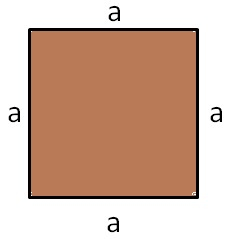# Find the area of a square of 9 cm

GIven: Square of the edge of 9 cm

To find: Area of the square

Solution:

Area of a square of side 'a' units is a$\times$a = a2 square units.

In the given square, the space shaded in brown is the area of the square.Area$=a^2$

= 9$\times$9

= 81 sq cm

Updated on: 10-Oct-2022

37 Views